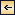Physical Properties of Sea Water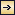### Transmission of Sound

Water is a very efficient medium for the transmission of sound, which travels more rapidly and with much less absorption of energy through water than through air. This characteristic has made possible the development of submarine acoustic methods that are of tremendous value in navigation. The most familiar use is in echo sounding, where the time required for an impulse to travel to the sea floor and back to the vessel is used as a measure of the depth. Horizontal sound transmission is used in radio-acoustic range finding, which is employed by surveying vessels in order to determine their location accurately when carrying on sounding operations out of sight of land. The position of the vessel is determined by measuring the time intervals required for the impulse of a bomb explosion to travel to two or more submarine sound detectors (hydrophones) at known locations, usually near shore. Sound transmission from subsurface bells has also been used as a navigational aid near lighthouses. Ultrasonic impulses (frequency above the range audible to the human ear) are largely directional, and many attempts have been made to develop instruments for locating icebergs and other navigational hazards in the path of a vessel. The practical aspects of this problem and the types of equipment used are too numerous to consider in this discussion, and reference is made to the Hydrographic Review (Monaco) as an excellent source of material on these problems.

75
COEFFICIENT OF THERMAL EXPANSION PER 1°C FOR SEA ICE (e × 104) (From Malmgren)
Salinity (‰) Temperature (°C)
−2 −4 −6 −8 −10 −12 −14 −16 −18 −20 −22
2………… −22.10 −4.12 −1.06 0.16 0.83 1.13 1.23 1.27 1.33 1.38 1.44
4………… −45.89 −9.92 −3.81 −1.37 −0.02 0.57 0.78 0.85 0.96 1.07 1.88
6………… −69.67 −15.73 −6.55 −2.90 −0.88 0.00 0.33 0.43 0.60 0.76 0.93
8………… −93.46 −21.53 −9.30 −4.43 −1.73 −0.57 −0.13 0.02 0.23 0.45 0.67
10………… −117.25 −27.34 −12.05 −5.95 −2.59 −1.13 −0.59 −0.40 −0.13 0.14 0.42
15………… −176.72 −41.85 −18.92 −9.78 −4.73 −2.54 −1.72 −1.45 −1.04 −0.63 −0.22

76

The velocity of sound in sea water is independent of the wave length except for impulses resulting from the detonation of relatively large amounts of explosives. Initially the impulse from such explosions may travel about 30 per cent faster than normal, apparently because of the tremendous energy involved.

The velocity of sound in a liquid may be computed from the elasticity and density: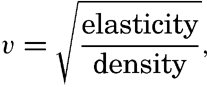[Equation]
but in practice it is more convenient to use the expression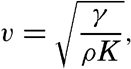[Equation]
where γ is the ratio of the specific heats, cp/cv (p. 62), ρ is the density, and K is the true compressibility. If ρ and K are in c.g.s. units, the velocity is in centimeters per second. The ratio γ is introduced because the sound impulse is a wave of compression and, hence, heats the water it passes through. The three variables, γ, ρ, and K, all change with temperature, salinity, and pressure, and therefore must be evaluated for any given set of conditions. For example, water of salinity 34.85 ‰ at 30° has a density of 1.021637 at atmospheric pressure (p = 0), and for these conditions γ = 1.0207 and K = 4.196 × 10−11. Hence,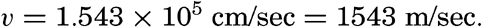[Equation]

77
By means of the above formula, suitable tables have been prepared that give the velocity of sound as a function of temperature, salinity, and pressure. The first practical tables were those of Heck and Service (1924) of the U. S. Coast and Geodetic Survey, but these have been superseded by the British Admiralty Tables prepared by Matthews (1927), which, although based on the same original data, are slightly more consistent. The variations in velocity as a function of temperature and salinity are shown in fig. 17. The effect of pressure, and hence depth, is shown in fig. 18. This effect is almost independent of the temperature and salinity, but the curve shown in the figure is actually for salinity 34.85 ‰ and 0°C. This salinity is the standard reference salinity used in these tables, and corrections due to salinity variations are given in the form of anomalies to be added to or subtracted from the standard values.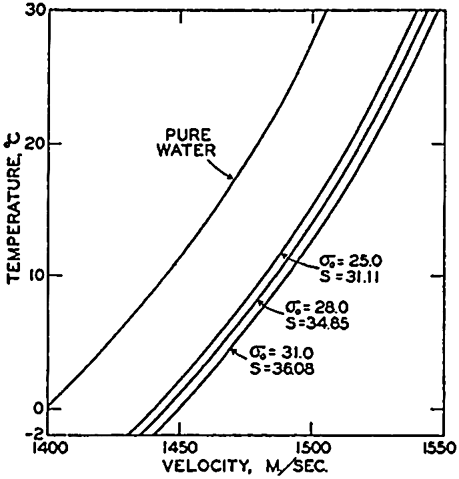#### Velocity of sound in pure water and in sea water at atmospheric pressure as a function of temperature and salinity.

[Full Size]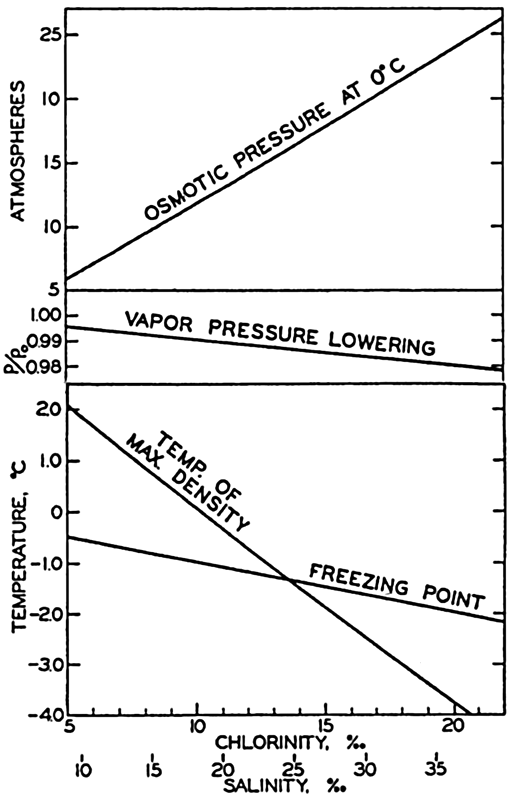#### Effect of pressure upon the velocity of sound in sea water of salinity 34.85 ‰ at 0°.

[Full Size]

If the velocity of the sound is known, it is possible to determine the wavelength of sound of different frequencies from the equation λ = υ/n, where λ is the wave length, υ is the velocity, and n is the frequency (table 20). Frequencies between about 25 and 10,000 vibrations per second are easily detected by the human ear, and the maximum sensitivity is at about 1000 per second. Ultrasonic waves of frequencies above those audible to the human ear have certain desirable properties that make them valuable in submarine acoustics, but, as will be shown later, their effective range is much less because their absorption is greater. The effective range has been increased by constructing sound emitters which for small wave lengths give a directional beam, somewhat

78
analogous to that of a searchlight. In practice the spread is about 10° to 15°. A directional beam serves not only to measure the distance to some reflecting body, but also to determine the direction to the body.

WAVE LENGTHS IN AIR AND WATER FOR SOUND OF DIFFERENT FREQUENCIES
Air Sea water Sea water
ϑ = 20°, S = 34.85 ‰ S = 34.85 ‰
Frequency p = 1 atm ϑ = 0°, p = 1 atm ϑ = 20°, p = 1 atm
v = 346 rn/sec v = 1445.2 m/sec v = 1518.5 m/sec
wave length, λ (m) wave length, λ (m) wave length, λ (m)
10 35.6 144.5 151.8
100 3.56 14.45 15.18
1,000 0.36 1.44 1.52
10,000 0.036 0.144 0.152
40,000 0.009 0.036 0.038
100,000 0.0036 0.0144 0.0152

In the absence of appreciable absorption and refraction the intensity of sound varies inversely as the square of the distance from the source. Owing to the viscosity of the water, a certain amount of the kinetic energy of the sound waves is converted into heat, and hence there is an absorption of sound somewhat analogous to that of light. The problem of absorption of sound in sea water has been discussed by Langevin (1924). The sound intensity of a plane sound wave decreases exponentially from I0, to Ix, by passage through a distance x. Therefore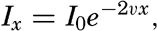[Equation]
where ν = 8π2μ/3λ2ρυ and corresponds to the absorption coefficient for radiant energy. The distance d over which the intensity is reduced to 1 /e (approximately 1/3) is therefore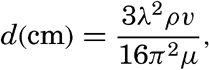[Equation]
where all values are in c.g.s. units. The quantities λ, ρ, and υ have already been defined, and μ is the dynamic viscosity. The ranges of ρ and v are small, and therefore d varies as λ2/μ. Hence the absorption increases rapidly with increasing frequency and somewhat with increasing viscosity, and is significant only for ultrasonic waves. According to Bergmann
79
(1939)
the absorption in water is much greater than is indicated by the equations above. Hartmann and Focke (1940) have obtained experimental data which indicate that the absorption is approximately a thousandfold larger. Whether absorption in the sea is as great or greater than that shown by these laboratory tests must await investigation in the field.

The velocity with which a sound wave travels through the water varies considerably with depth. Hence a “beam” of ultrasonic waves may be refracted when transmitted in a quasi-horizontal direction. Generally the velocity decreases with increasing depth in the upper layers and the beam is bent downward. Studies made by the U. S. Coast and Geodetic Survey (Swainson, 1936) have shown that a sound impulse may travel directly to the hydrophone, or may reach the hydrophone after having been reflected one or more times at the surface and the bottom. In many cases it was possible to distinguish several different “rays” which were received after different intervals. The direct transmission could be obtained only at distances less than 20 km and when the depth to the bottom was about 2000 m. The velocity of the direct wave impulse corresponded to that computed from the temperature and salinity, but those wave impulses which were reflected showed an apparent “horizontal” velocity less than the theoretical because of the longer paths traveled. This empirical horizontal velocity depends upon the distance between the vessel and the hydrophone, the depth to the bottom, the bottom profile, the physical properties of the water, and so forth.#### Vertical distributions of temperature and salinity off southern California at 32°57′N and 122°07′W, the computed velocity of sound, and the mean sounding velocity.

[Full Size]

As stated previously, the vertical velocity is a function of the depth and the distribution of temperature and salinity. Most sonic depth-finding instruments are adjusted for a constant “sounding velocity,” usually 800 to 820 fathoms per second (1463 to 1500 m/sec). In certain cases it is desirable to correct the readings to true depths. This can be done if the distributions of temperature and salinity are known. For different areas of the oceans and for different depths, the British Admiralty Tables contain “mean sounding velocities”; that is, mean velocities from the surface to the stated depth. In general, these first decrease somewhat with depth, because the effect of decreasing temperature dominates, but at greater depths they increase again as the pressure

80
effect becomes dominant. In fig. 19 are shown the vertical temperature and salinity distributions at a station off the coast of southern California together with the corresponding velocity of sound at all depths as computed from the British Admiralty Tables. The mean sounding velocity is also shown. The latter decreased from 1503 m/sec at the surface to a minimum of 1484 m/sec for depths between 800 and 1800 m, and then increased again to 1496 m/sec at 4000 m.Physical Properties of Sea Water